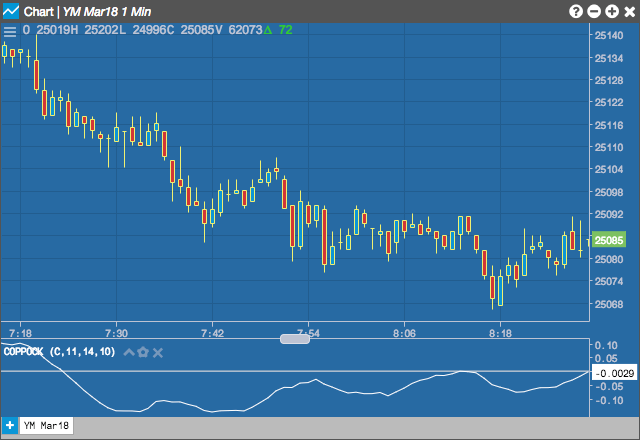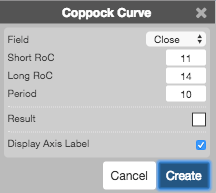Charts

# Coppock Curve (CC)

The Coppock Curve indicator tries to identify buy signals in a market by looking at market activity on a monthly time scale. It adds the rates of change for a short and a long period of months and then smooths by another period's weighted moving average. Typically, a potential buy signal occurs when the indicator is below 0 and then rises above it.## Configuration Options• Field: Price or combination of prices to use as the base for average calculations. Possible values include:
• Open
• High
• Low
• Close
• HL/2 $$\left ( \frac{High + Low}{2} \right )$$
• HLC/3 $$\left ( \frac{High + Low + Close}{3} \right )$$
• HLCC/4 $$\left ( \frac{High + Low + Close + Close}{4} \right )$$
• OHLC/4 $$\left ( \frac{Open + High + Low + Close}{4} \right )$$
• Short RoC: Number of periods in the short rate-of-change indicator.
• Long RoC: Number of periods in the long rate-of-change indicator.
• Period: Number of bars to use in the calculations.
• Color Selectors: Colors to use for graph elements.
• Display Axis Label: Whether to display the most recent value on the Y axis.

## Formula

$Coppock = WMA_\text{period}\;of\;(ROC_\text{Long RoC periods}+ROC_\text{Short RoC periods})$

where:

• WMA is the weighted moving average.
• ROC is the rate of change indicator.